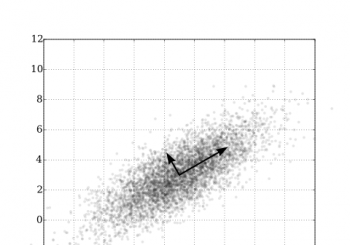# Statistics

## Fitting the Weibull Distribution

The Weibull distribution is widely used in reliability analysis, hazard analysis, for modeling part failure rates and in many other applications. The NMath library currently includes 19 probably distributions and has recently added a fitting function to the Weibull distribution class at the request of a customer. The Weibull probability distribution, over the random variable x, has two para...

## Principal Components Regression: Part 3 – The NIPALS AlgorithmPrincipal Components Regression: Recap of Part 2 Recall that the least squares solution to the multiple linear problem is given by(1) $latex \hat{\beta} = (X^T X)^{-1} X^T y$ And that problems occurred finding when the matrix(2) was close to being singular. The Principal Components Regression approach to addressing the problem is to replace in equation (1) with a better conditioned...

## Principal Components Regression: Part 2 – The Problem With Linear Regression

This is the second part in a three part series on PCR, the first article on the topic can be found here. The Linear Regression Model Multiple Linear Regression (MLR) is a common approach to modeling the relationship between one or two or more explanatory variables and a response variable by fitting a linear equation to observed data. First let’s set up some notation. I will be rather brief, ass...